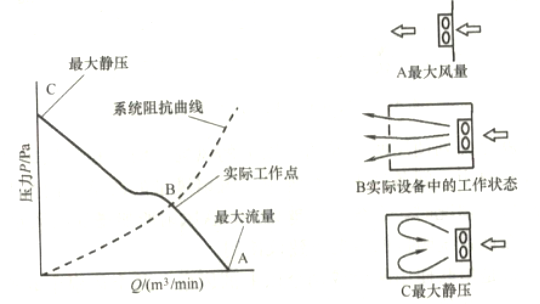0755-23327569

1主要元器件工作温度要求；

2产品使用环境温度要求：

3）产品发热总量。

P =QATρCp                               2-1

Q=P/(TρCp                           2-2

Q=P/(TρCp)=P/(1.078T)                      2-3

Q=P/(21.56)                            2-4

Q=P/(21.56*1000)                        2-5

Q=P/(21.56*1000)*60=P/359                 2-6

Q=P/(21.56*1000)*2118.88=0.0983P(CFM)     2-7

Q=35*0.0983=3.4CFM

Q=180*0.0983=17.7CFM2-1  某风扇PQ线和工作点信息示意

QQ3260145042

E-MAILKira@ruid-tech.com